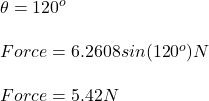## A wire 2.80 m in length carries a current of 5.20 A in a region where a uniform magnetic field has a magnitude of 0.430 T. Calculate the mag

Question

A wire 2.80 m in length carries a current of 5.20 A in a region where a uniform magnetic field has a magnitude of 0.430 T. Calculate the magnitude of the magnetic force on the wire assuming the following angles between the magnetic field and the current.

in progress 0
6 months 2021-08-05T21:23:27+00:00 1 Answers 9 views 0

## Answers ( )

1. Question:

A wire 2.80 m in length carries a current of 5.20 A in a region where a uniform magnetic field has a magnitude of 0.430 T. Calculate the magnitude of the magnetic force on the wire assuming the following angles between the magnetic field and the current.

(a)60 (b)90 (c)120

(a)5.42 N (b)6.26 N (c)5.42 N

Explanation:

From the question

Length of wire (L) = 2.80 m

Current in wire (I) = 5.20 A

Magnetic field (B) = 0.430 T

Angle are different in each part.

The magnetic force is given by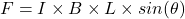So from data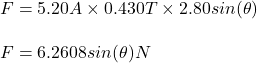Now sub parts

(a)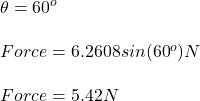(b)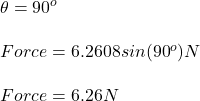(c)